Open in App
Not now

# How to create a Venn Diagram in R ?

• Last Updated : 17 Jun, 2021

Venn diagram is the graphical representation of sets used for showing the relationship between them. Through the use of Venn diagram one can highlight the differences as well as similarities between elements of sets. Venn diagram is also known as Logic diagram or set diagram. We use circles to represent sets that can be intersecting or overlapping or non-intersecting, based on relationship between them. There are a number of operations we can perform using Venn diagram, but the major among them are: Union, Intersection, and Complement.

• Union: Union of sets refers to the combined elements of all sets.
• Intersection: Intersection of sets is the set of elements containing the common element of both sets. When there is no common element, the intersection will give a null value.
• Complement: The complement of a set is the set of all elements in the universal set other than the set itself. The complement of an empty set is the universal set.

In this article, we are going to implement Venn diagram using R programming language.

### Method 1:Using “VennDiagram R Package”

We need to install and load the package first:

Syntax:

install.packages(“VennDiagram”)

library(“VennDiagram”)

For creating a Venn diagram for pair sets or for triple sets or for any number of sets, there is only a slight difference in the syntax. For example, we use keyword “pairwise” for creating a Venn diagram with two sets, and for three sets we use “triple” keyword.

• For two sets:

Syntax:

grid.newpage()

draw.pairwise.venn(area1,area2,cross.area,col,fill)

• For three sets:

Syntax:

grid.newpage()

draw.triple.venn(area1,area2, area3,n12,n23,n13, n123,category)

Parameters:

• area1: The size of first set
• area2: The size of second set
• area3: The size of third set
• cross.area: The size of the intersection between the sets
• n12: The size of intersection between the first and second set
• n23: The size of intersection between the third and second set
• n13: The size of intersection between the first and third set
• n123: The size of intersection between all the three sets
• col: A vector giving the colours of the circle’s circumference
• fill: A vector giving the colours to circle’s area
• category: A vector of strings giving the category names of the sets

In order to create a Venn diagram using this package install and load the package in the editor.  Add a new plotting page using grid.newpage( ). Then, insert the data for Venn diagram using the above syntax. For one set use draw.single, two sets use draw.pairwise, three sets use draw.triple, four sets use draw.quad and five sets use quintuple.

Example 1:

## R

 `# load Venn diagram package``library``(``"VennDiagram"``)`` ` `# move to new plotting page``grid.newpage``()`` ` `# create pairwise Venn diagram``draw.pairwise.venn``(area1=20, area2=45,cross.area=10,``                   ``category=``c``(``"Mango"``,``"Banana"``),fill=``c``(``"Red"``,``"Yellow"``))`

Output: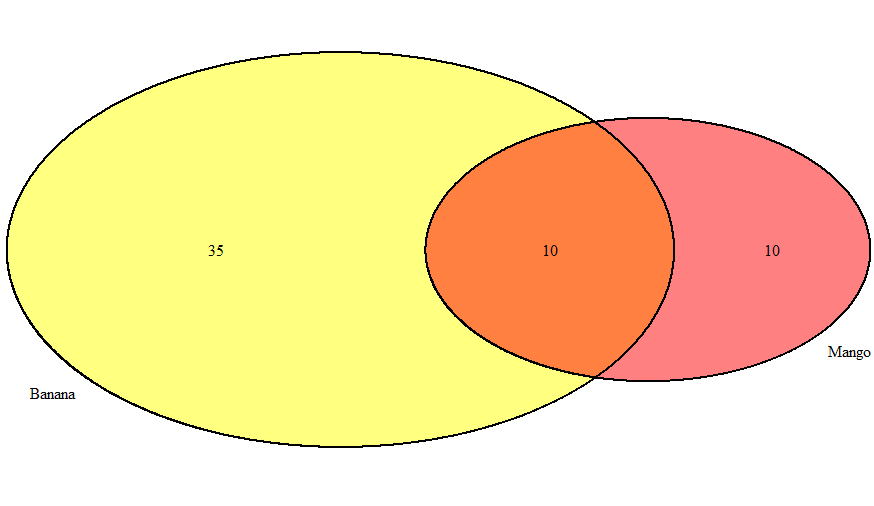Example 2:

## R

 `# load Venn diagram package``library``(``"VennDiagram"``)`` ` `# move to new plotting page``grid.newpage``()`` ` `# create Venn diagram with three sets``draw.triple.venn``(area1=40, area2=15, area3=10, ``                 ``n12=5, n23=12, n13=4, n123=2, ``                 ``category=``c``(``"Science"``,``"Economics"``,``"English"``),``                 ``col=``"Red"``,fill=``c``(``"Green"``,``"Yellow"``,``"Blue"``))`

Output: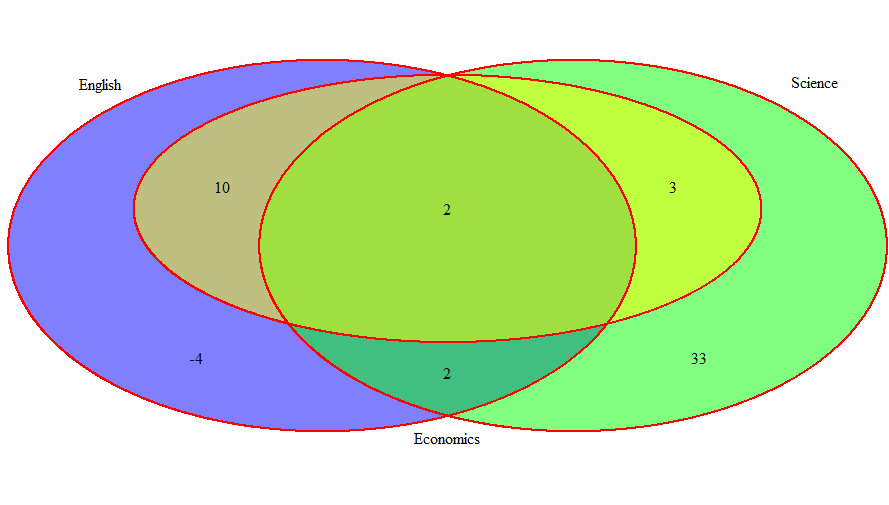Example 3:

## R

 `# load Venn diagram package``library``(``"VennDiagram"``)`` ` `# move to new plotting page``grid.newpage``()`` ` `# create Venn diagram with four sets``draw.quad.venn``(area1=72, area2=86, area3=50, ``               ``area4 =52, n12=44, n23=38, n13=27, ``               ``n14= 32,n24=32, n34=20, n123=18, ``               ``n124=17, n234=13, n134=11, n1234=6, ``               ``category=``c``(``"Cricket"``,``"Football"``,``"Badminton"``,``"Table Tennis"``),``               ``col=``"Green"``,fill=``c``(``"Red"``,``"Pink"``,``"Blue"``,``"Orange"``),lty=``"dashed"``)`

Output: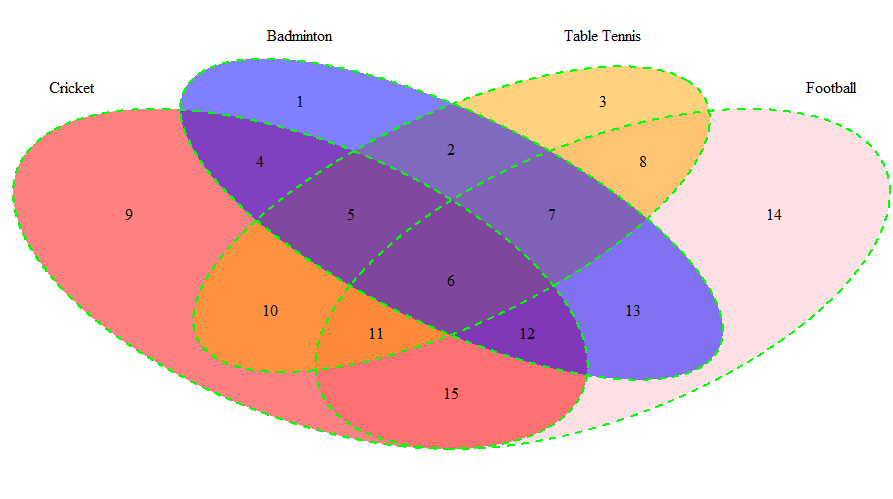### Method 2: Using “GGVENN”  R package

First, we need to install this package and load it in order to make it usable.

install.packages(“ggvenn”)

library(“ggvenn”)

ggvenn plots venn diagram as an independent function. It supports both data frame and list as input.

Syntax:

ggvenn (data, show_elements, show_percentage, fill_color, stroke_color, stroke_linetype)

Parameters:

• data: A data frame or list as input data
• show_elements: Shows set elements instead of count
• show_percentage: Show percentage for each set
• fill_color: Fill colours in circles
• stroke_color: Stroke colour for drawing circles
• stroke_linetype: Line type for drawing circles

This approach is simple and only data to be plotted, needs to be passed.

Example 1:

## R

 `# load ggvenn package``library``(``"ggvenn"``)`` ` `# use list as input``A <-``list``(``'India'``=``c``(1,3,5,7),``'USA'``=``c``(1,6,5))`` ` `# create venn diagram and display all the sets``ggvenn``(A)`

Output: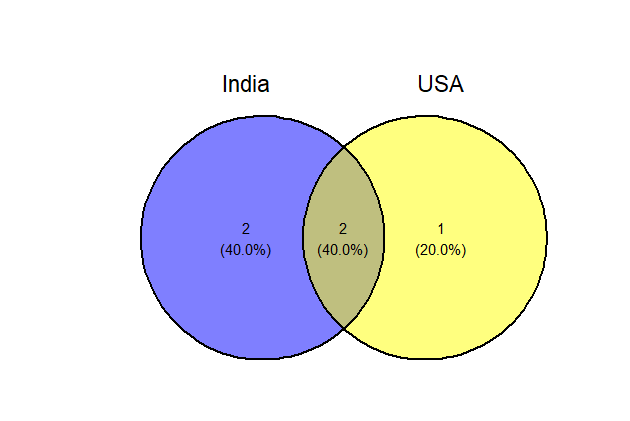Example 2:

## R

 `# load ggvenn package``library``(``"ggvenn"``)`` ` `# use data frame as input``M <-``tibble``(value=``c``(1,3,2,7,5),``'TEA'``=``c``(``TRUE``,``FALSE``,``TRUE``,``FALSE``,``FALSE``),``           ``'COFFEE'``=``c``(``TRUE``,``TRUE``,``FALSE``,``FALSE``,``TRUE``),  ``           ``'JUICE'``=``c``(``TRUE``,``FALSE``,``FALSE``,``TRUE``,``TRUE``))`` ` `# create Venn diagram and display all sets``ggvenn``(M)`

Output: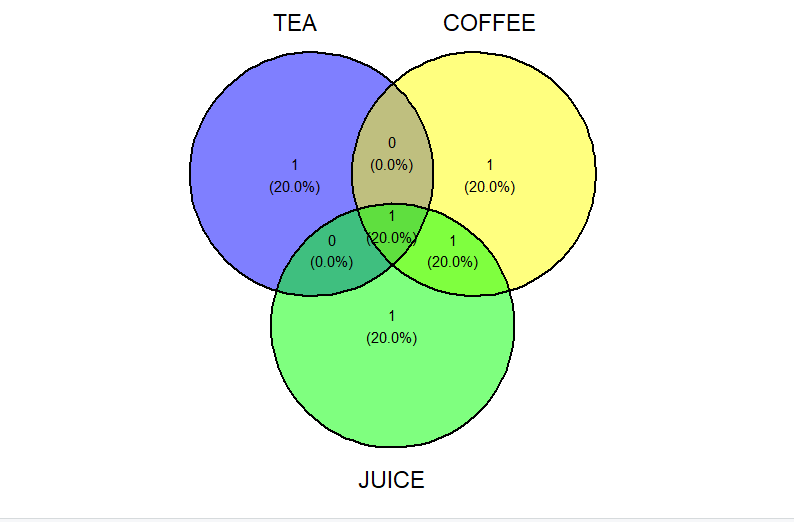Example 3:

## R

 `# load ggvenn package``library``(``"ggvenn"``)`` ` `# use list as input``D <-``list``(``'Autumn'``=``c``(2,3,1),``'Winter'``=``c``(4,7,9,1),``         ``'Summer'``=``c``(3,7,2,5),``'Spring'``=``c``(2,5,9,1))`` ` `# creating venn diagram for four sets``# and displaying only two sets``ggvenn``(D,``c``(``"Autumn"``,``"Spring"``),show_percentage=``FALSE``,``       ``fill_color=``c``(``"red"``,``"orange"``))`

Output: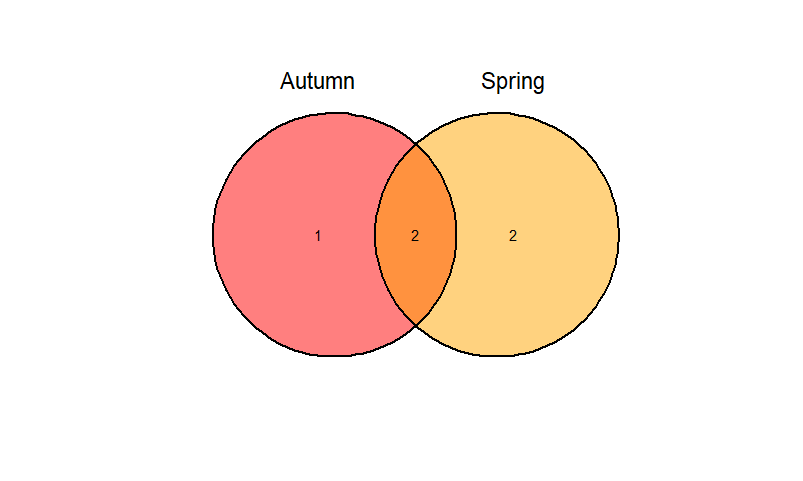Example 4:

## R

 `# load ggvenn package``library``(``"ggvenn"``)`` ` `# use list as input ``H <-``list``(``'Bus'``=``c``(6,7,3),``'Truck'``=``c``(4,3,9),``         ``'Cycle'``=``c``(10,3,2,8),``'Car'``=``c``(7,5,4,3))`` ` `# create customised venn diagram``ggvenn``(H,show_elements=``TRUE``,stroke_color=``"Red"``,``       ``stroke_linetype=``"solid"``)`

Output: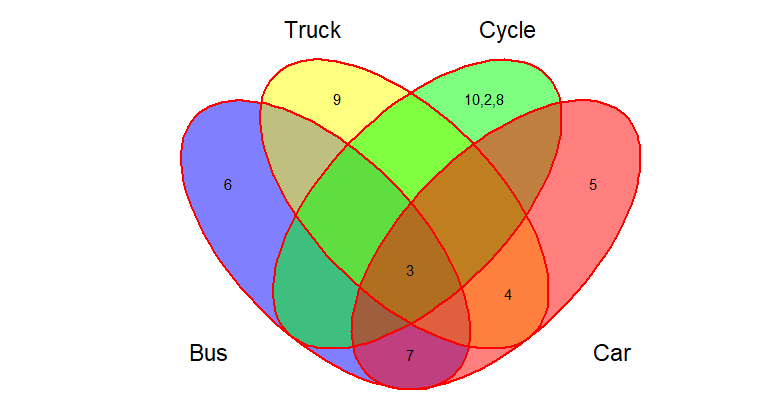### Method 3: Using “GPLOTS” R package

The gplots package provides Venn diagrams for up to five sets. The venn( ) function accepts either a list of sets as an argument, or it takes a binary matrix, one column per set, indicating for every element, one per row, the membership with every set. The main page of venn( ) lists options to change the appearance of the plots, e.g., the names of the sets may be omitted and sizes changed. However, there is ample opportunity to extend the functionality of this package.

As usual, the first step is to install and load the package:

install.packages(“gplots”)

library(“gplots”)

We simply pass the input as a parameter in venn( ) function.

Example 1:

## R

 `# load gplots package``library``(``"gplots"``)`` ` `# use list as input``x <-``list``(``'Plants'``=``c``(6,7,8),``'Animals'``=``c``(7,8,3,4))`` ` `# create Venn diagram with two sets``venn``(x)`

Output: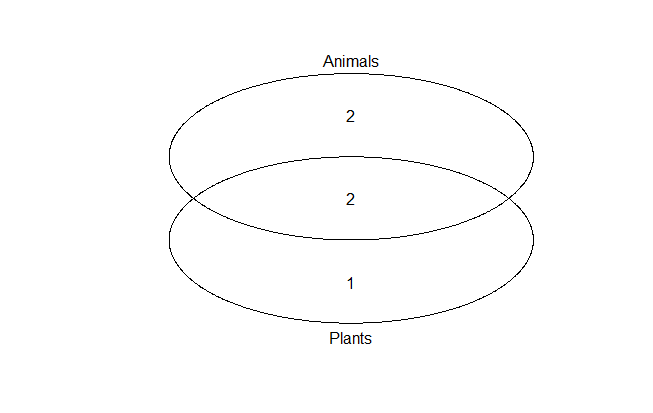Example 2:

## R

 `# load gplots package``library``(``"gplots"``)`` ` `# creating venn diagram by passing ``# list as direct parameter``venn``(``list``(YouTube=1:6,Netflix=3:8,Instagram=``c``(5,8:12)))`

Output: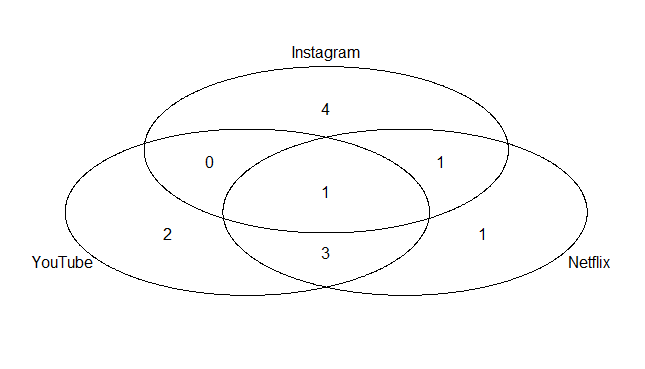### Method 4: Using “GGVENNDIAGRAM” R package

‘ggVennDiagram’ returns structured data that can be used to plot Venn. The first step to make use of this package is to install and load it.

install.packages(“ggVennDiagram”)

library(“ggVennDiagram”)

Here also we will simply pass the parameter in ggVennDiagram( ) function.

Syntax:

ggVennDiagram(data, label_alpha, category.names, show_intersect, set_color)

Parameters:

• data: Input in form of list
• label_alpha: Remove the background of region labels
• category.names: Give names to sets.
• show_intersect: If true, text can be visualized
• set_color: Color of set labels

For this to work for, first install and load ggVennDiagram Package and give input as list. Then, use the above syntax for creating Venn Diagram and for customizing Venn diagram use other parameters.

Example 1:

## R

 `# load ggVennDiagram Package``library``(``"ggVennDiagram"``)`` ` `# use list as an input``x <-``list``(``'C++'``=``c``(9,3,5,2),``'Java'``=``c``(7,8,4,3),``         ``'Python'``=``c``(11,2,4,5,8),``'Ruby'``=``c``(3,8))`` ` `# creating Venn diagram and displaying ``# all sets``ggVennDiagram``(x)`

Output: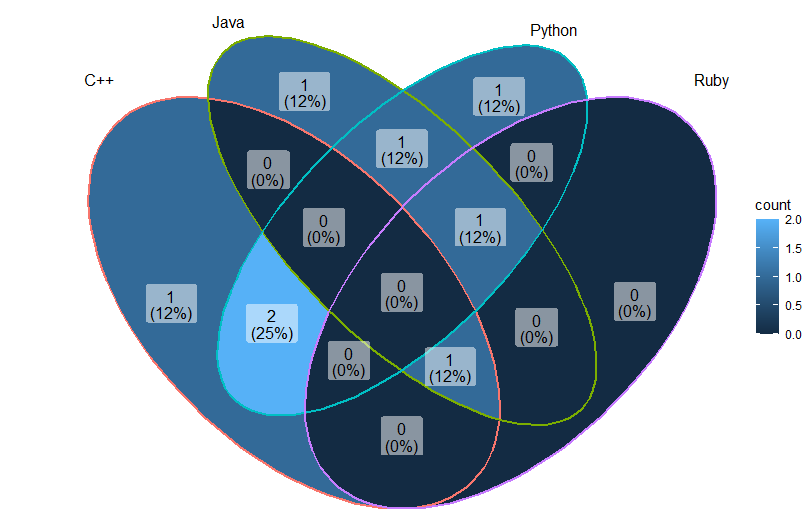Example 2:

## R

 `# load ggVennDiagram Package``library``(``"ggVennDiagram"``)`` ` `# use list as input``M <-``list``(``'Rose'``=``c``(2,6),``'Lily'``=``c``(5,3,9,2),``         ``'Sunflower'``=``c``(3,10,2),``'Lotus'``=``c``(5,7,8))`` ` `# creating Venn diagram with four sets``# but displaying only first two``ggVennDiagram``(M[1:2],set_color=``"Red"``,label_alpha=0,``              ``show_intersect=``FALSE``)`

Output: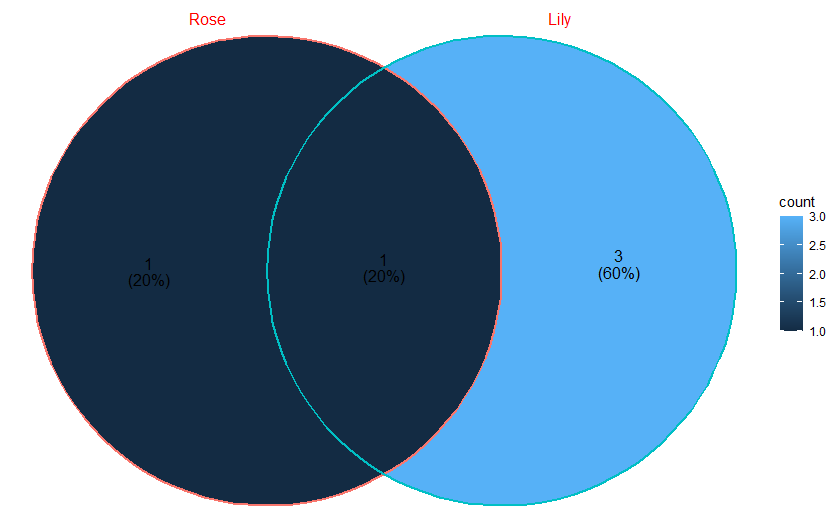Example 3:

## R

 `# load ggVennDiagram Package``library``(``"ggVennDiagram"``)`` ` `# use list as input``D<-``list``(A=``c``(5,6,8),B=``c``(1,9,8,2),C=``c``(8,9,4,7,11))`` ` `# creating Venn diagram with three sets``# but displaying only last two``ggVennDiagram``(D[2:3],category.names=``c``(``"BREAKFAST"``,``"LUNCH"``))`

Output: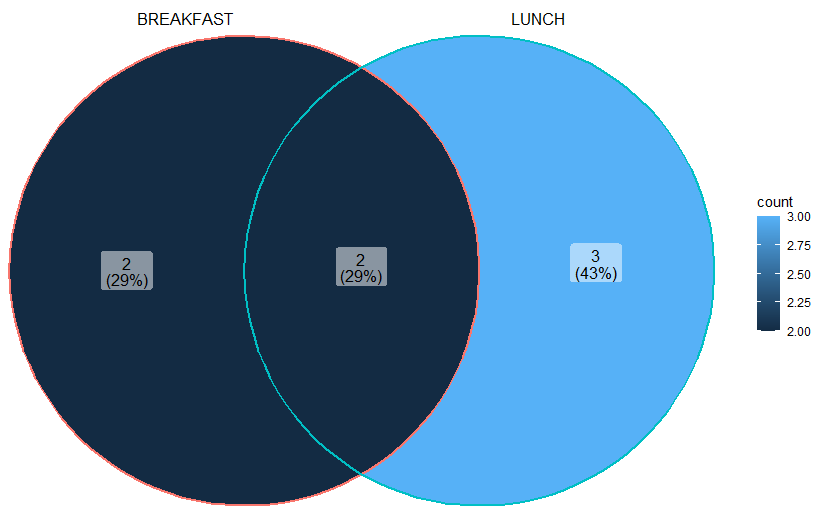My Personal Notes arrow_drop_up